# Fraction Calculator

Simplify
Backspace
A/C
0
Whole Numbers
1
2
3
4
5
6
7
8
9
0
Numerators
1
2
3
4
5
6
7
8
9
0
Denominators
1
2
3
4
5
6
7
8
9
÷
x
+
=

Fraction calculator for multiplication, division, addition, and subtraction of two or more fractions and whole numbers. It can process multiple fractions and whole numbers at once. Then it displays the step by step solutions of whatever operation it has processed. Sometimes it is called fraction solver or mixed number calculator. As of now it can compute up to ten both fractions and mixed numbers. It is useful for all students in all grade levels. It can be used as a reference to all math teachers and even those professionals who often use fractions in their workplace or in their homes.

## How To Use Fraction Calculator?

This fraction calculator has been designed for easy use.

• Press the whole number button if your fraction has a whole number or you can directly press the numerator button if you don’t need whole number. You cannot press denominator button if you have not pressed whole number or denominator button. This means that you need to press the whole number or numerator button first. Once numerator button is pressed, you can no longer press whole number button. You can only press whole number button again if you delete the numerator by pressing the backspace button. Zeroes should not be pressed first. Zeroes will be pressed after non-zero numbers are pressed.
• Press denominator button for your denominator. Once pressed, you cannot press whole number or numerator button again. You can only press numerator button if you delete the denominator by pressing the backspace button.
• Select any operation you want.
• Press equal button if you are done with your fraction. The solution will be displayed above.
• Press Backspace if you want to delete one number at a time.
• Press AC button to reset the fraction calculator.
• As of now, this calculator is limited only to 10 fractions.

## Addition and Subtraction of fractions using calculator

Addition and subtraction of fraction follows the same rules. They must have the same denominators to process the selected operation. You can add or subtract the two fractions if they have the same denominator, if not; you have to create a common denominator before adding or subtracting them. When using fraction calculator, you just have to press the numerator button and followed by denominator button to make it a fraction. Then you have to select the operation you want either plus button or minus button. Then you create your second fraction. The same process will be used to the third, fourth or any numbers of fractions you want. Then hit the equal button for the computation. Watch the video below for the demo.

## Multiplication and Division of fractions using calculator

For multiplication of fractions, you just have to multiply the numerator for the first fraction to the numerator of the second denominator and multiply the denominator of the first fraction to the denominator of the second fraction. When using calculator, you just have to create your first fraction followed by multiplication sign, followed by your next fraction. Watch video below.

For division of fractions, the standard rule is to invert first the second fraction and then proceed with multiplication. In fraction calculator, you will no longer have such pain in the neck. You just enter you first fraction followed by division sign and then your next fraction. Watch video below.

## Addition, Subtraction, Multiplication and Division of mixed numbers using fraction calculator

When dealing with mixed numbers, the important point to remember if you use fraction calculator is never forget to enter the whole numbers. The whole number buttons in the calculator is larger than the numerator and denominator buttons. You only need to press first the numerator button followed by fraction then you can proceed to any operation you want.

## Fraction Operation Rules

### Adding Two Like Fractions

Like fractions are fractions that have the same denominators. To add fractions with the same denominator add its numerator. For example, 2/5 + 1/5 = 3/5.

### Adding Unlike Fractions

Fractions that have different denominators are unlike fractions. To add unlike fractions you need to make them have a common denominator. The simplest way to do it is by following the steps below.

1. Multiply the numerator of the first fraction to the denominator of the second fraction. The result would be the first fraction numerator.
2. Multiply the denominator of the first fraction to the denominator of the second fraction. The result would be the new denominator of the first fraction.
3. Multiply the numerator of the second fraction with the denominator of the first fraction. The result would be the new numerator of the second fraction.
4. Multiply the denominator of the second fraction with the denominator of the first fraction. The result is now the new denominator of the second fraction.

For example: 2/3 + 3/5.

1. 2 x 5 = 10.
2. 3 x 5 = 15.
3. 3 x 3 = 9.
4. 5 x 3 = 15.

The new fraction are 10/15 and 9/15.
10/15 + 9/15 = 19/15.
The new fraction is 19/15.

### Subtraction Of Two Like Fractions

To subtract fractions with the same denominator, just subtract the numerator of the second fraction from the numerator of the first fraction. Example: 4/6 – 3/6 = 1/6.

### Subtractions of Two Unlike Fractions

Follow the rules of Adding Two Unlike fractions but instead of adding it, just subtract it after they have the same denominator.

### Multiplying Two Fractions

The rule of multiplying two fractions is straightforward. Multiply the numerator of first fraction to the numerator of the second fraction and multiply the denominator of the first fraction to the denominator of the second fraction. Example: 2/3 x 1/5 = 2/15.

### Dividing Two Fractions

To divide two fractions, you must first invert the second fraction then start multiplying the two fractions. Example: 2/3 divided by 1/5 = 2/3 x 5/1 = 10/3.

### What Are Fractions?

Fractions are parts of a whole. For example there is one cake for five children. So the cake is divided into five parts. Each child would receive one part of the cake. The fraction would be 1/5. Each child will receive 1/5 of cake.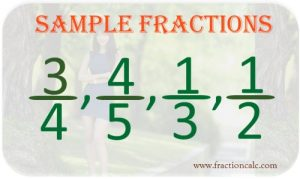### Parts of Fraction

There are two parts of fraction. The upper half part is called numerator. The lower half part is called denominator. Numerator is the part of the whole where it is being used or currently being dealt with.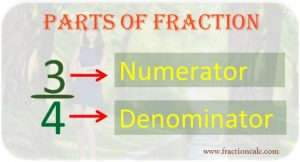### Types of Fractions

There are three types of fractions: proper fraction, improper fraction, and mixed numbers.

Proper fraction is a fraction where its numerator is always lesser than its denominator.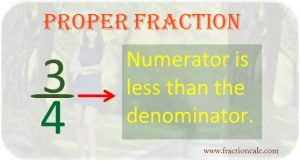Improper fraction is a fraction where its numerator is equal or greater than its denominator.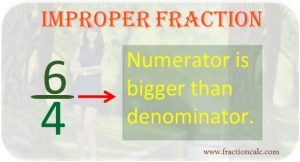Mixed Number represents whole numbers plus a fraction.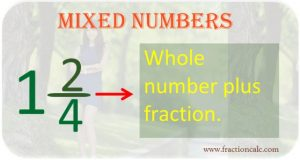### How To Change Improper Fraction to Mixed Number

When you reduce improper fraction to its lowest term, you need to change it to mixed number. This is done by dividing the numerator to denominator. The quotient will be the whole number. The remainder will be the new numerator and the denominator will remain as is.

### How To Change Mixed Number To Improper Fraction

In dividing or multiplying mixed numbers, you need it to change into improper fraction. This is done by multiplying the whole number to denominator then adds the current numerator. The result will be the new numerator and the denominator will remain as is.

### What Are Equivalent Fractions?

Equivalent fractions are fractions that have different numerator and denominators but have the same value such as 1/2, 2/4, 7/14, 8/16, 10/20, 20/40, and 50/100.

### Comparing Fractions

For fractions that have the same denominators, the fraction that has the biggest numerator is the larger fraction than the fraction that has smaller numerator.
For fractions that have the same numerators, the fraction with the biggest denominator is smaller than the fraction that has smaller denominator.

### Simplifying Fractions

From the topic above, we already know that there are equivalent fractions-fractions that are the same in value even if they have different numerators and denominators. Simplifying fraction means that using the smallest numerator and denominator but the same value. The fraction is in its simplest form when there is no common factor for numerator and denominator. For example, instead of using 7/14, we can use ½ which is the simplest form.

### Greatest Common Factor

Greatest common factor is a largest number used to divide both numerator and denominator to get the simplest form of a fraction. For example, for fraction 12/30, the largest number to divide both the numerator and denominator is 6. By dividing it to 6, you will arrive to its simplest form which is 2/5.

## How Fraction Calculator Was Created?

When I was a student, I have this math subject. One of the topics was about fraction. Although this topic is challenging, it greatly astonished me why it is hard to determine if the solution is right or wrong. You have to review it a number of times in order to be certain that your solution is right. It did not happen on me only. I found out that most of the students experienced the same. So, from there on, I dream that for one way or another, I will help them. I will help them to make sure that their solution is right without going through a lot of reviews. That is why I created this fraction calculator. This fraction calculator was created as a reference or a guide only to make sure that the student arrive the correct answer from their fraction problems. From The Founder of FractionCalc.com